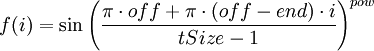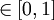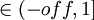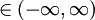# SP - Sinebell Apodization (nmrPipe function)

## Overview

Sine-bell apodization used in nmrPipe is defined i-th point as follows:$f(i) = \sin\left( \frac{\pi \cdot off + \pi \cdot (off-end) \cdot i}{tSize-1} \right)^{pow}$

where value of off corresponds to value of -off parameter, end - to -end parameter, pow - to -pow parameter. tSize is automatically read from the data file (it's header). Meanings of parameters are described below.

## Parameters

 parameter type and range of values meaning -off real number,$\in [0,1]$ starting offset if sine bell in units of π -end real number,$\in (-off,1]$ ending offset if sine bell in units of π -pow real number,$\in (-\infty,\infty)$, but typical values are 1.0 and 2.0 exponent of the apodization function -c,-start,-size,-one,-hdr,-inv extra parameters that apply to all available window functions; -c is the most frequently used one